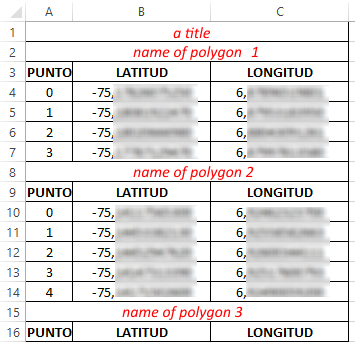# Importing data from excel to create polygons

8259
2
01-17-2016 02:12 AMNew Contributor II

Hello,

I am new to ARCGIS and had a question about the best way to import polygon data from a csv into ARCGIS.

I am able to import a csv file which has lat/long points to create an XY event layer, and then create a shapfile, etc. I was wondering what the best way would be to do this for polygon data. I am going to have a csv file which has the lat/long of the vertexes of the polygons and some of the polygon areas will be square (and have four vertexes), however some of the polygons will have more complex shapes and may have 10 or more vertexes. I am aware of the Points to Line and Feature to Polygon tools, which I think will allow me to import the vertexes to create one polygon.

However I have 200 or so polygons to import into my map, so this will take a long time. Is there an easy way to bring in the batch data points to create all of my polygons at once? Do I need to get some add-in tool?

Thanks very much

Brendan

2 RepliesbyMVP Legendary Contributor

My first question would be what is the source of the data?  Recreating polygons from a stream of points is definitely possible in a variety of ways, but the most elegant will entail python programming.  If your data came from  a gps unit, then it is possible to recreate polylines/polygons if you have the track information and the tracks represent closed-loops.  So some background might save some time

if you have a list of lists of point objects (ie xy pairs) you can construct polygons using the following.  The construction of the point objects will depend on the nature of your input data, and is left for elsewhere

output_shp - an output file name including path

SR - a spatial reference object

pnts - a list of point objects (nested, a list for each polygon inside a list)

```def output_polygons(output_shp,SR,pnts):
"""produce the output polygon shapefile"""
assert (SR != None) and (SR.type=='Projected'), msg
polygons = []
for pnt in pnts:                           # create the polygon geometry
polygons.append(arcpy.Polygon(arcpy.Array([arcpy.Point(*xy) for xy in pnt]),SR))
if arcpy.Exists(output_shp):               # overwrite any existing versions
arcpy.Delete_management(output_shp)
arcpy.CopyFeatures_management(polygons, output_shp)  ```byEsri Esteemed Contributor

Last year I created a simple tool to allow some coworkers to convert data in Excel format to polygons.

It is based on the following format:They save the Excel file as textfile (tab delimited)

The parameters are as follows:

Code:

```# -*- coding: utf-8 -*-
#-------------------------------------------------------------------------------
# Name:        PuntosPoligonosTXT.py
# Purpose:    covertir puntos a poligonos
#
# Author:      xbakker
#
# Created:    10/04/2015
#-------------------------------------------------------------------------------

import arcpy
import os

def main():
import sys
import traceback

try:
arcpy.env.overwriteOutput = True

# first parameter is txt file
txt = arcpy.GetParameterAsText(0)

# forth parameter (provide default NOMBRE)
fld_name = arcpy.GetParameterAsText(1)

# second parameter is output fc
fc_out = arcpy.GetParameterAsText(2)

# third parameter is spatial reference
sr = arcpy.GetParameter(3)

# create empty output fc
ws_name, fc_name = os.path.split(fc_out)
geomtype = "POLYGON"
arcpy.CreateFeatureclass_management(ws_name, fc_name, geomtype, spatial_reference=sr)

# start insert cursor
flds = ("SHAPE@", fld_name)
with arcpy.da.InsertCursor(fc_out, flds) as curs:

cnt = 0
nombre = ""
first_point = None
lst_pnt = []

with open(txt, 'r') as f:
cnt += 1
if cnt > 1:
line = line.replace('\n','')
lst_line = line.split('\t')
a = lst_line
if a.isdigit():
# point
if bln_start:
first_point = GetPoint(line)
pnt = GetPoint(line)
lst_pnt.append(pnt)
else:
pnt = GetPoint(line)
lst_pnt.append(pnt)
bln_start = False
else:
if a[:5].upper() == 'PUNTO':
pass
else:
if first_point != None:
lst_pnt.append(first_point)

if len(lst_pnt) > 2:
# create previous polygon and write to fc
polygon = arcpy.Polygon(arcpy.Array(lst_pnt), sr)
curs.insertRow((polygon, nombre, ))
lst_pnt = []
nombre = line.strip()
bln_start = True

if first_point != None:
lst_pnt.append(first_point)
polygon = arcpy.Polygon(arcpy.Array(lst_pnt), sr)
curs.insertRow((polygon, nombre, ))

except:
tb = sys.exc_info()
tbinfo = traceback.format_tb(tb)
pymsg = "Errores de Python:\nTraceback info:\n" + tbinfo + "\nError Info:\n" + str(sys.exc_info())
msgs = "Errores de ArcPy:\n" + arcpy.GetMessages(2) + "\n"